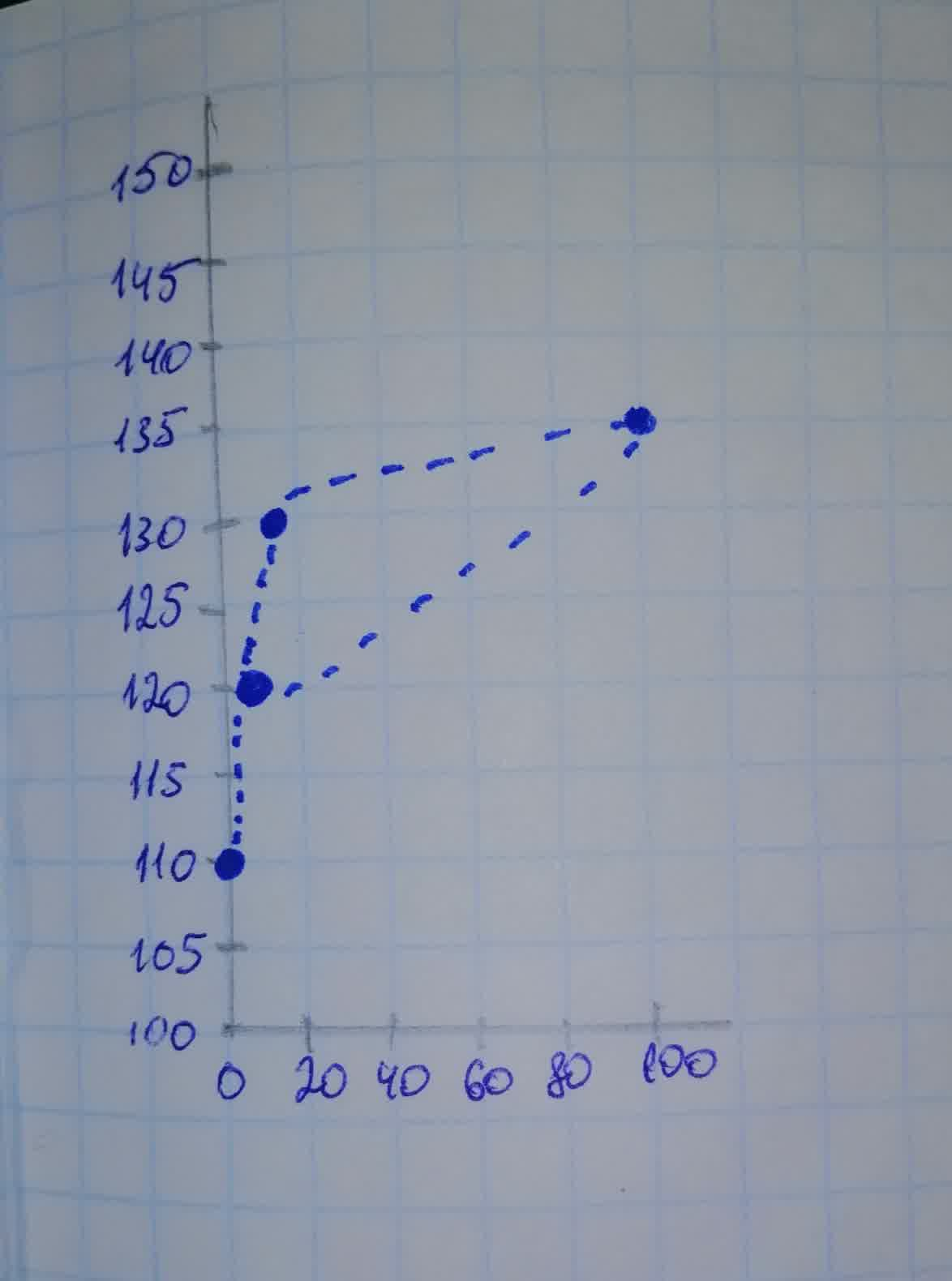Presenting data in the form of table. For the data set shown by the table.Create a scatter plot for the data.Use the scatter plot to determine whether an exponential function, a logarithmic function, or a linear function is the best choice for modeling the data.UkusakazaL 2021-08-08

Presenting data in the form of table. For the data set shown by the table, Solve,
a) Create a scatter plot for the data.
b) Use the scatter plot to determine whether an exponential function, a logarithmic function, or a linear function is the best choice for modeling the data. (If applicable, you will use your graphing utility to obtain these functions.)
$$\begin{array}{|c|c|}\hline \text{Intensity (wattd per}\ meter^{2}) & \text{Loudness Level (decibels)} \\ \hline 0.1\text{(loud thunder)} & 110 \\ \hline 1\text{(rock concert, 2 yd from speakers)} & 120 \\ \hline 10 \text{(jackhammer)} & 130 \\ \hline 100 \text{(jet take off, 40 yd away)} & 140 \\ \hline \end{array}$$

• Questions are typically answered in as fast as 30 minutes

Solve your problem for the price of one coffee

• Math expert for every subject
• Pay only if we can solve itquestion2answer

Step 1
a) We can draw scatter plot using Excel as follows,
Enter the data in Excel and select it.
Go to insert $$\displaystyle\rightarrow$$ scatter $$\displaystyle\rightarrow$$ select 1st scatter plot $$\displaystyle\rightarrow$$ add trendline to the plot $$\displaystyle\rightarrow$$ Tick on display equation on plot and display R square on the plot.
We get result as follows,Step 2
b) From the above scatter plot, we can observe that, the regression equation for logarithmic model is,
$$\displaystyle\hat{{{y}}}={4.3429}{\ln{{\left({x}\right)}}}+{120}$$
and $$\displaystyle{R}^{{{2}}}={1}$$
Since, the value of $$\displaystyle{R}^{{{2}}}={1}$$ for Logarithmic function, the logarithmic function is the best choice for modeling the data.